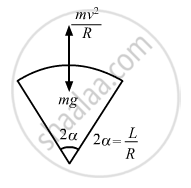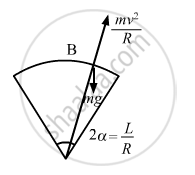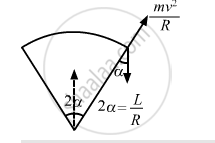Share

# A Motorcycle Has to Move with a Constant Speed on an Over Bridge Which is in the Form of a Circular Arc of Radius R and Has a Total Length L. What Can Its Maximum Velocity Be for Which the Contact - Physics

ConceptCircular Motion

#### Question

A motorcycle has to move with a constant speed on an over bridge which is in the form of a circular arc of radius R and has a total length L. Suppose the motorcycle starts from the highest point.(a) What can its maximum velocity be for which the contact with the road is not broken at the highest point? (b) If the motorcycle goes at speed 1/√2 times the maximum found in part (a), where will it lose the contact with the road? (c) What maximum uniform speed can it maintain on the bridge if it does not lose contact anywhere on the bridge?

#### Solution

R = Radius of the bridge
L = Total length of the over bridge

(a) At the highest point:
Let m be the mass of the motorcycle and v be the required velocity.$\text{mg }= \frac{\text{mv}^2}{\text{R}}$

$\Rightarrow \text{v}^2 = \text{Rg}$

$\Rightarrow \text{v} = \sqrt{\text{Rrg}}$

$\left( b \right) \text {Given :}$

$\text{v} = \left( \frac{1}{\sqrt{2}} \right)\sqrt{\text{Rg}}$Suppose it loses contact at B.

$\text {At point B, we get : }$

$\text{mg}\cos\theta = \frac{\text{mv}^2}{R}$

$\Rightarrow \text{v}^2 = \text{Rg}\cos\theta$

$\text {Putting the value of v}, \text {we get : }$

$\sqrt{\left( \frac{Rg}{2} \right)^2} = Rg\cos\theta$

$\Rightarrow \frac{Rg}{2} = Rg\cos\theta$

$\Rightarrow \cos\theta = \frac{1}{2}$

$\Rightarrow \theta = 60^\circ = \frac{\pi}{3}$

$\because \theta = \frac{L}{R}$

$\therefore L = R\theta = \frac{\pi R}{3}$

So, it will lose contact at a distance $\frac{\pi R}{3}$  from the highest point.

(c) Let the uniform speed on the bridge be v. The chances of losing contact is maximum at the end bridge. We have :$\alpha = \frac{L}{2R}$

$\text{So}, \frac{\text{mv}^2}{R} = \text{mg}\cos\alpha$

$\Rightarrow v = \sqrt{\text{gRcos}\left( \frac{L}{2R} \right)}$

Is there an error in this question or solution?

#### APPEARS IN

Solution A Motorcycle Has to Move with a Constant Speed on an Over Bridge Which is in the Form of a Circular Arc of Radius R and Has a Total Length L. What Can Its Maximum Velocity Be for Which the Contact Concept: Circular Motion.
S